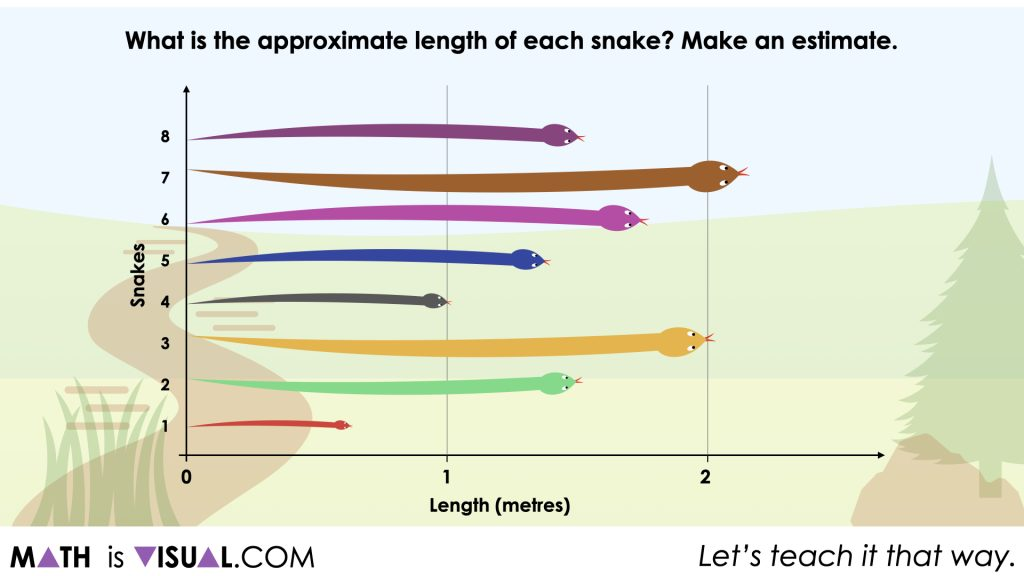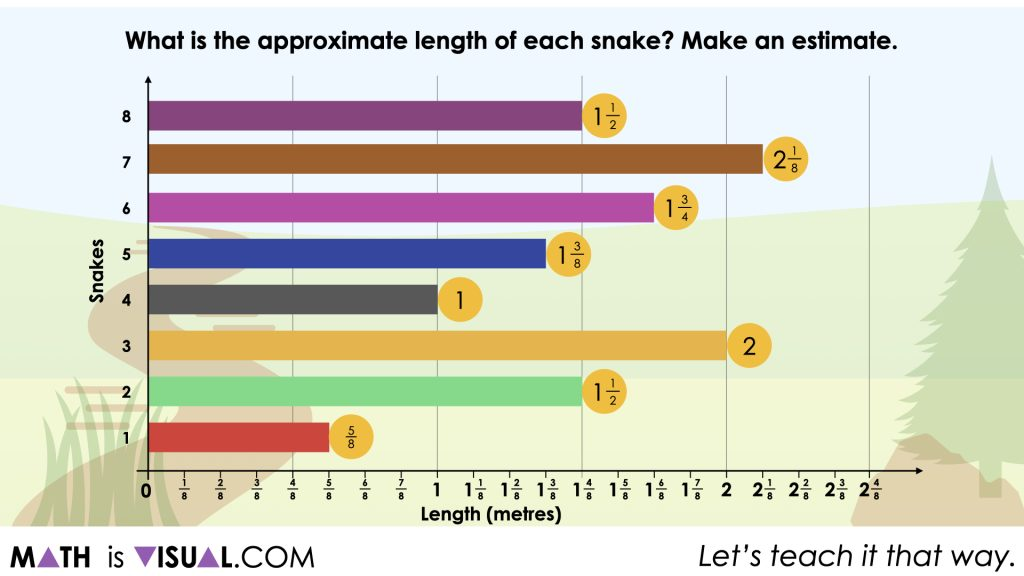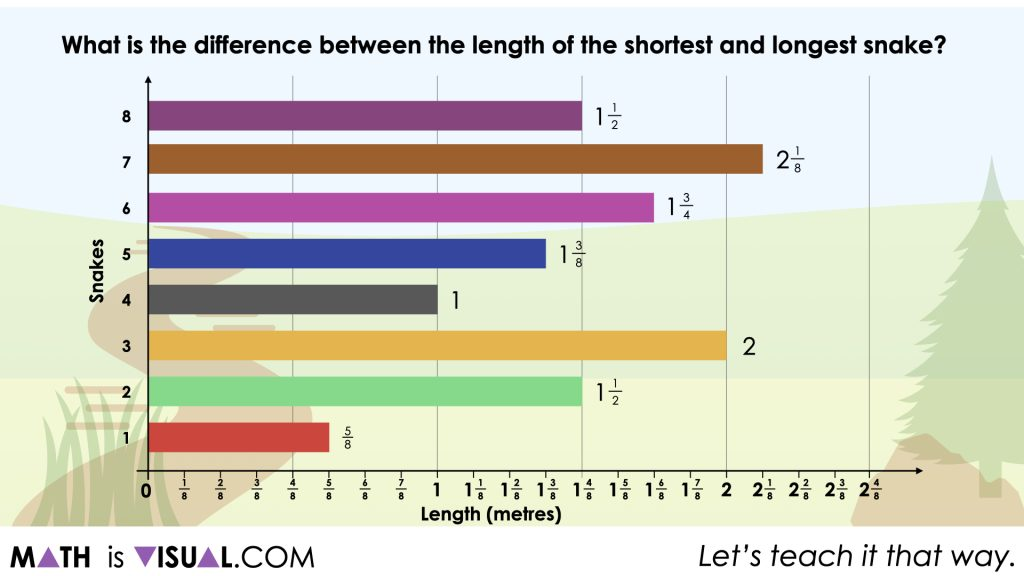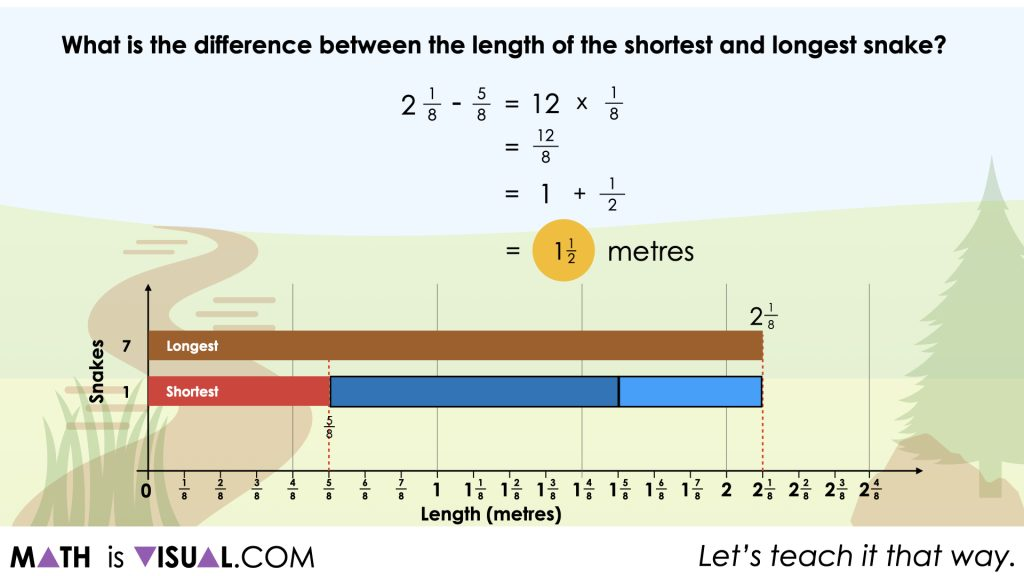# Add and Subtract Fractions Through Data Literacy

Visual number talk prompts building fluency and flexibility adding and subtracting fractions through a categorical data context.

## In This Set of Visual Number Talk Prompts…

Students will explore displayed categorical data sets with measurements in fractions of a unit and determining the difference between two data points. This visual math talk serves to develop a deeper understanding of the following big ideas.

• There are different types of data;
• Categorical data refers to a data type that can be identified based on the names or labels given to them;
• Categorical data is either nominal or ordinal;
• Bar graphs are used to compare and contrast numbers, frequencies or other measures of distinct categories of data;
• The numerical values of a data set need to be considered when a scale is chosen;
• A fraction can represent a part of a whole;
• A structure of subtraction is comparison or difference.

## String of Related Problems

Present the following series of Visual Math Talk Prompts to students involving a bar graph displaying the length of eight fox snakes in metres.

Students will be asked to draw conclusions about the data on display.

Some questions students will encounter and you should consider asking include:

1. What can you tell me about the length of these snakes?
2. Why do you think they selected a bar graph?
3. What would happen to the bars if we change the scale to one-tenth of a metre?
4. What is the length of the shortest snake?
5. What is the length of the longest snake?
6. What is the difference between the length of the shortest and longest snake?

## Visual Number Talk Prompt #01

Students Prompt:

What is the approximate length of each snake?

Make an estimate.Some students may immediately notice that they can measure with certainty the length of both snakes 3 and 4 at 2 metres and 1 metre in length respectively. However, the remaining 6 snakes will require them to make fractional estimates based on their spatial reasoning and understanding of fractional quantities.

Give students an opportunity to estimate and discuss with their neighbours. You might also encourage them to use the template included in the editable Lesson Template and Consolidation Prompts document for students to partition the snakes into halves, fourths, eighths and/or thirds, sixths, etc. to help them improve their precision.

After students have updated their estimates and shared with the group, you can share the actual lengths of each snake and celebrate students who had reasonable estimates relatively close to the actual lengths shown.## Visual Number Talk Prompt #02

Students Prompt:

What is the difference between the length of the shortest and longest snake?

Craft a convincing argument without the use of a calculator.It is likely that most students will be able to identify the shortest (snake 1) and longest (snake 7), however determining an accurate difference in length may be more challenging.

However, encourage students to compare the length of both snakes and even come up with the closest number of whole metres to represent the difference.

• the same length as snake 1?
• 2 metres longer?
• 3 metres longer?
• What seems reasonable?

After students share their thinking here, ask them to be more precise.

Promote students leveraging a model of their choice to help justify their thinking. Since the bar graph has a nice linear model (number line) at the bottom, some students might do “jumps” in full metres and fractional metres until they reach the length of snake 7 to determine a more precise length.

Highlight student strategies and models leveraged and also model the symbolic representation(s) they are sharing so they can make connections between the visual and symbolic/abstract representations.## Want to Explore These Concepts & Skills Further?

Four (4) additional number talk prompts are available in Day 2 of the Evergreen problem based math unit that you can dive into now.

Why not start from the beginning of this contextual 8-day unit of real world lessons from the Make Math Moments Problem Based Units page.

Did you use this in your classroom or at home? How’d it go? Post in the comments!

Math IS Visual. Let’s teach it that way.#### kylepearce3

•Nse says:
•kylepearce3 says: